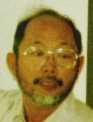Mathematical Physics and Quantum Field Theory
Electron. J. Diff. Eqns., Conf. 04, 2000, pp. 121-145.

### A partial solution for Feynman's problem -- A new derivation of the Weyl equation Atsushi Inoue

Abstract:
Associating classical mechanics to a system of partial differential equations, we give a procedure for Feynman-type quantization of a "Schrödinger-type equation with spin." Mathematically, we construct a "good parametrix" for the Weyl equation with an external electromagnetic field. Main ingredients are (i) a new interpretation of the matrix structure using superanalysis and (ii) another interpretation of the method of characteristics as a quantization procedure of Feynman type.

Published July 12, 2000.
Mathematics Subject Classifications: 35F10, 35L45, 35Q40, 70H99, 81S40.
Key words: Quantization, good parametrix, spin, superanalysis.

Show me the PDF file (262K), TEX file, and other files for this article.Atsushi Inoue Department of Mathematics Tokyo Institute of Technology 2-12-1 Oh-okayama, Meguro-ku Tokyo 152-0033, Japan e-mail: inoue@@math.titech.ac.jp